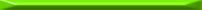### Introduction

Although I've written some recursive-descent (RD) parsers by hand, the theory behind them eluded me for some time. I had a good understanding of the theory behind bottom-up LR parsers, and have used tools (like Yacc and PLY) to generate LALR parsers for languages, but I didn't really dig into the books about LL.

This week I've finally decided to understand what's going on. I tried to write a simple RD parser in Python (previously I've written RD parsers in C++ and Lisp), and ran into a problem which got me thinking hard about LL parsers. So, I've opened the Dragon Book, and now I know much more about LL(1), LL(k), predictive, recursive-descent parsers with and without backtracking, and what's between them.

This article is a summary of my findings, written for myself to read in a few months when I forget it :-)

### Recursive descent parsers

From Wikipedia:

A recursive descent parser is a top-down parser built from a set of mutually-recursive procedures (or a non-recursive equivalent) where each such procedure usually implements one of the production rules of the grammar. Thus the structure of the resulting program closely mirrors that of the grammar it recognizes.

RD parsers are the most general form of top-down parsing, and the most popular type of parsers to write by hand. However, being so general, they have several problems, like requiring backtracking (which is difficult to code correctly and efficiently).

Usually, it is enough to use less general and powerful parsers for all practical needs, like parsing programming languages (and domain specific languages). This is where LL parsers come in.

### LL parsers

An LL parser is a top-down parser for a subset of the context-free grammars. It parses the input from Left to right, and constructs a Leftmost derivation of the sentence (hence LL, compared with LR parser). The class of grammars which are parsable in this way is known as the LL grammars.

LL parsers are further classified by the amount of lookup they need. LL(1) parsers require 1 character of lookup, LL(k) require k, and so on. Usually, LL(1) is enough for most practical needs.

LL parsers are also called predictive, because it's possible predict the exact path to take by a certain amount of lookup symbols, without backtracking.

### The example

This week I tried to construct a RD parser for this simple calculator grammar:

```<expr>      :=  <term> + <expr>
|   <term> - <expr>
|   <term>
<term>      :=  <factor> * <term>
<factor> / <term>
<factor>
<factor>    :=  <number>
|   <id>
|   ( <expr> )
<number>    :=  \d+
<id>        :=  [a-zA-Z_]\w+
```

This grammar is LL(1) and hence parseable by a simple predictive parser with a single token lookahead. However, I then tried to add the following rule to allow input of commands into an interactive calculator prompt:

```<command>   :=  <expr>
|   <id> = <expr>
```

With this rule added, the grammar is no longer LL(1), because looking at the first token I can't say which one of the two options of <command> it is. In order to be able to differentiate between an assignment and a single expression, I must see the = token, and for this I need to see 2 tokens forward, and not just one. So, this grammar turns into a LL(2).

LL(2) grammars are much more difficult to code by hand than LL(1) grammars, and they are also much more difficult to turn into code automatically by parser generators. This is probably why for most languages LL(1) suffices.

### LL parser generators

Unlike LR parsers, for which everyone uses parser generators , LL parsers are commonly written by hand. It even appears that some of the most popular compilers (such as GCC) use hand-written RD parsers to parse whole languages like C. As with anything, you get maximal flexibility and efficiency when you hand-code something, as you're not constrained by the limitations of the tools and libraries you're using.

Indeed, writing a simple predictive parser as a set of mutually recursive routines is simple, and can also be very educational. If you have a very small parsing task to perform, perhaps you'll be better off hand-coding a RD parser.

However, automatic tools for generating LL parsers exist. The most popular are probably ANTLR and Boost.Spirit. I haven't tried them, but both are widely used to write complex parsers. Both have a clear advantage over hand-written parsers - they can generate parsers with any lookup length, guessing the required length from the grammar. Hand-written parsers, as I mentioned earlier, get much more complex for any k > 1.

### Left recursion

Had my expr rule been written like this:

```<expr>      :=  <expr> + <term>
|   <expr> - <term>
|   <term>
```

It would have been left recursive, because the non-terminal expr appears as the first (leftmost) symbol in its own production. Since RD parsers work top-down, to recognize <expr> it has to first recognize <expr>, but for that it again has to recognize <expr> and so on, ad infinitum. This infinite recursion is the reason why RD parsers can't handle left recursion.

Left recursion can also be indirect:

```<a>   :=  <b> <x>
|   <c>
<b>   :=  <a> <y>
|   <d>
```

Here we can have the infinite derivation: <a> -> <b> <x> -> <a> <y> <x> and so on.

Techniques exist to remove left recursion from some grammars. For more information see this. The grammar shown in the example above had left-recursion removed from it .

### Code

A simple recursive descent parser for a calculator, written in Python, can be downloaded here. It also includes a fairly generic Lexer class that implements regex-based tokenization of a string.Since LR parsers are table-based are too tedious and unwieldy to write by hand.
  Which, however, has left it with a slight operator associativity problem. Finding it is left as an exercise for the reader).